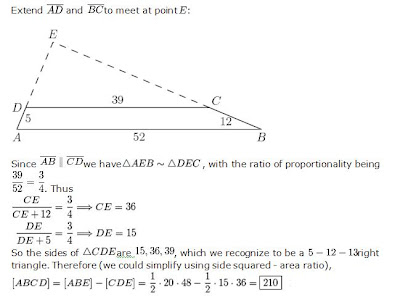### Solve it - Question 11

Dear All

One more very interesting question for all of you:

In trapezium ABCD with bases AB and CD, AB = 52 , BC = 12, CD = 39, and DA = 5. Find the area of ABCD.

Here is the Solution:### Solve it - Question 10

Dear All

Writing after so long... I was busy doing many other assignments.

So, here is a question for you :)

Question :

Twenty matchsticks of equal length are placed to form a triangle. Find the total number of different triangles that can be made with a perimeter of 20 matchsticks?